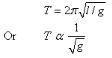×
Get Full Access to Physics: Principles With Applications - 6 Edition - Chapter 11 - Problem 7q
Get Full Access to Physics: Principles With Applications - 6 Edition - Chapter 11 - Problem 7q

×

# If a pendulum clock is accurate at sea level, will it gainISBN: 9780130606204 3

## Solution for problem 7Q Chapter 11

Physics: Principles with Applications | 6th Edition

• Textbook Solutions
• 2901 Step-by-step solutions solved by professors and subject experts
• Get 24/7 help from StudySoup virtual teaching assistantsPhysics: Principles with Applications | 6th Edition

4 5 1 373 Reviews
10
4
Problem 7Q

Problem 7Q

If a pendulum clock is accurate at sea level, will it gain or lose time when taken to high attitude? Why?

Step-by-Step Solution:

Step 1 of 1

The equation for pendulum motion is:As we gain altitude the value of the constant of gravity ‘g’ decreases.

If a pendulum clock that is accurate at sea level is taken to a high altitude it will slow down. The time period is inversely proportional to the square root of ‘g’.

When ‘g’ decreases Time period ‘T’ increases.

So the clock will slow down.

Step 2 of 1

##### ISBN: 9780130606204

Physics: Principles with Applications was written by and is associated to the ISBN: 9780130606204. This full solution covers the following key subjects: accurate, attitude, clock, gain, high. This expansive textbook survival guide covers 35 chapters, and 3914 solutions. Since the solution to 7Q from 11 chapter was answered, more than 1797 students have viewed the full step-by-step answer. The full step-by-step solution to problem: 7Q from chapter: 11 was answered by , our top Physics solution expert on 03/03/17, 03:53PM. The answer to “If a pendulum clock is accurate at sea level, will it gain or lose time when taken to high attitude? Why?” is broken down into a number of easy to follow steps, and 21 words. This textbook survival guide was created for the textbook: Physics: Principles with Applications, edition: 6.

## Discover and learn what students are asking

Statistics: Informed Decisions Using Data : Testing the Significance of the Least-Squares Regression Model
?Suppose a least-squares regression line is given by y = 4.302x - 3.293. What is the mean value of the response variable if x = 20?

Unlock Textbook Solution